Question

What is the length of side x in a 30-60-90 triangle where one side is the square root of 3 and the other side is unknown?

1.Tryphena

The length of side x in a 30-60-90 triangle is 2√3.

Step-by-step explanation:

The numbers 30-60-90 are angles, so we need to find the side x of a right triangle with the following information:

θ: is one angle of the right triangle = 30°

α: is the other angle of the right triangle = 60°

a: is one side of the right triangle = √3

b: is the other side of the right triangle =?

x: is the hypotenuse of the right triangle =?

The length of the hypotenuse can be found by Pitagoras: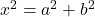(1)

So, we need to find the side “b”. We can calculate it with the given angles.

From the side “a” we have: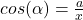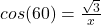(2)

From the side “b”: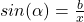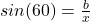(3)

Now, we can calculate “b” by dividing equation (3) by equation (2).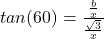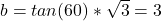Finally, we can find the length of the hypotenuse with equation (1):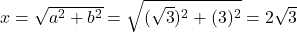Therefore, the length of side x in a 30-60-90 triangle is 2√3.

I hope it helps you!